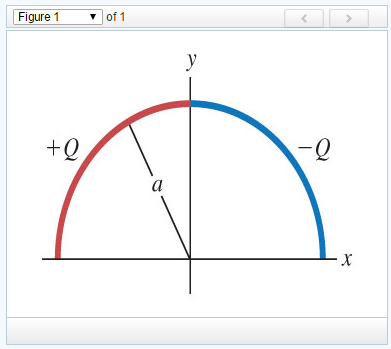# magnitude of the net electric field in a semicircle

A semicircle of radius a is in the first and second quadrants, with the center of curvature at the origin. Positive charge +Q is distributed uniformly around the left half of the semicircle, and negative charge −Q is distributed uniformly around the right half of the semicircle in the following figure.(Figure 1)

Part A What is the magnitude of the net electric field at the origin produced by this distribution of charge? Express your answer in terms of the variables Q, a, constant π, and electric constant ϵ0.

options: +x direction −x direction +y direction −y direction another direction

Part B What is the direction of the net electric field at the origin produced by this distribution of charge?